# Magnetic moment of a revolving electron, and Bohr magneton, class 12

•
•
•
•
•
•
•
•

In this article, we will discuss and derive an expression for the magnetic moment of an electron that is revolving around a nucleus. So without any delay. Let’s get started…

According to Neil Bohr – The negatively charged particle of an atom i.e electron revolves around the positively charged nucleus along a circular path with the radius r. As we know that, in any conductor, electrons are responsible for the creation of electric current because the motion of free electrons in the conductor produces the electric current.

If we follow this fact, then we will find that a revolving electron around the positively charged nucleus will also produce electric current because the electron is moving in a closed circular path around the nucleus, and as we know that motion of electric charge produces an electric current, so it does.

## Magnetic moment of a revolving electronFig. 1, Magnetic moment of a revolving electron. source: sarthaks econnect

Let a electron of massis revolving around a nucleus in a circular path of radius. Ifis the electronic charge and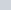is the time period of the revolution then current produced due to this motion is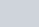Ifis the orbital velocity of the electron, then-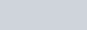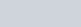Due to the orbital motion of the electron, it seems like that the current so produced is flowing through the circular loop and its path acts like a current loop, and we know that the magnetic dipole moment of a current-carrying loop is given as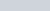which we have discussed the previous article.

So the orbital magnetic moment of a revolving electron in a circular path is given by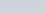where A is the area of the circular orbit. Substituting the value of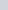in this equation, we get-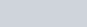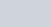Ifis the mass of the electron, then multiply numerator and denominator of the above equation by the mass () of the electron, after multiplying we get-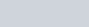Look the expression, it is nothing but the orbital angular momentum (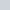) of the electron about the central nucleus.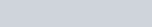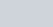The magnetic moment per angular momentum is a constant term which is given as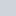is called the gyromagnetic ratio, whose value is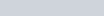. According to the Neil Bohr- angular momentum is quantised and only has the set of discrete values.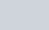Here, n is a natural number and h is Planck’s constant whose value is 6.626 × 10-34 Js. Substituting the expression of quantised angular momentum described by Neil Bohr in the above equation in place of, we get-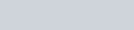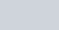The minimum value of the orbital magnetic moment is given when the value ofwill be lowest i.e n = 1.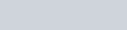If you put the value of,, andthen you will get the lowest value of the magnetic moment (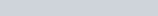).

This lowest value of the magnetic moment of the revolving electron is called the Bohr magneton. So we have discussed the orbital magnetic moment of a revolving electron but an electron also possesses a magnetic moment due to its spin in addition to the magnetic moment due to its orbital motion. As a result, an electron’s resultant magnetic moment is given by the vector sum of its orbital and spin magnetic moments.

Watch this video for more understanding.

Stay tuned with Laws Of Nature for more useful and interesting content.